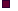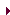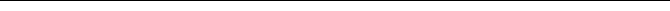Magnus Österholm
PublicationPublicationsCVResearchGo back

 Title: Läsa matematiska texter: Förståelse och lärande i läsprocessen [Reading mathematical texts: Understanding and learning in the reading process] Type: Licentiate thesis Fulltext: Has been published electronically through Linköping University Electronic Press Other: Information from Libris (Swedish university libraries)
 Abstract: The focus of this thesis is the reading of mathematical texts, especially how and what you can understand and learn from reading. The main interest is the reading process, that is, the reading itself and what you understand after reading a text. The main purpose is to study the reading of mathematical texts in particular, in order to test and develop an existing general theory of reading comprehension. An essential part is to study how the use of symbols in mathematical texts can affect the reading process. The thesis consists of theoretical discussions about the reading of mathematical texts and an empirical study among students from the Swedish upper secondary school and from the university. A study of existing literature that deal with properties of mathematical texts constitute a starting point for the theoretical discussions. In particular, the reading of mathematical symbols and algebraic expressions is discussed. The empirical study, which includes 106 participants, used three different texts: one history text about the Russian revolution and two mathematical texts about group theory. The same information about groups is included in both mathematical texts, but one of the texts uses mathematical symbols in the presen­tation while the other does not use symbols at all. Each participant read one of the mathematical texts and the history text, and after each text they got to answer questions about the content of the text. The group of participants who read the mathematical text without symbols has a better result on the questions about the text than the group of participants who read the text with symbols. This seems to be caused by an inability to articulate the symbols and by the fact that the ability to decode a text does not seem to be used in the same way for the text with symbols as it is for the history text and the mathematical texts without symbols. Thus, the reading of mathe­matical texts with symbols is a rather special activity and there might be a need for learning how to read such texts. On the other hand, there seems to be many similarities between the reading of the mathematical text without symbols and the historical text. Therefore, the main factor of the texts influencing the reading process is not the content of the text but the form of the text, that is, how the content is presented. In the theoretical discussions, some suggestions are presented on how the reading of mathematical texts with symbols can be included in the general theory of reading comprehension. In general there is no reason to consider the reading of mathematical texts as a special kind of reading process, different from the reading of other types of texts. Thus, the general theory of reading comprehension can function as a theoretical framework also for the comprehension of mathematical texts, possibly with the suggested additions about mathematical symbols.Magnus ÖsterholmMain pagePublicationsCVResearchUmeå UniversityUmeå School of EducationFaculty of Science andTechnologyDepartment of Scienceand Mathematics EducationUmeå MathematicsEducation Research CentreMid Sweden UniversityFaculty of Science,Technology and MediaDepartment of Mathematicsand Science EducationMonash UniversityFaculty of EducationInclusive MathematicsEducation Research GroupLinköping UniversityThe Institute of TechnologyDepartment of MathematicsContact for this page: magnus.osterholm@umu.se